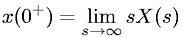Equations > Signal Processing > Laplace Transform Properties > Laplace transform initial value theorem

### Laplace transform initial value theoremLatex Code:

MathML Code:

 $x\left({0}^{+}\right)=\underset{s\to \infty }{lim}\mathrm{sX}\left(s\right)$

MathType 5.0: